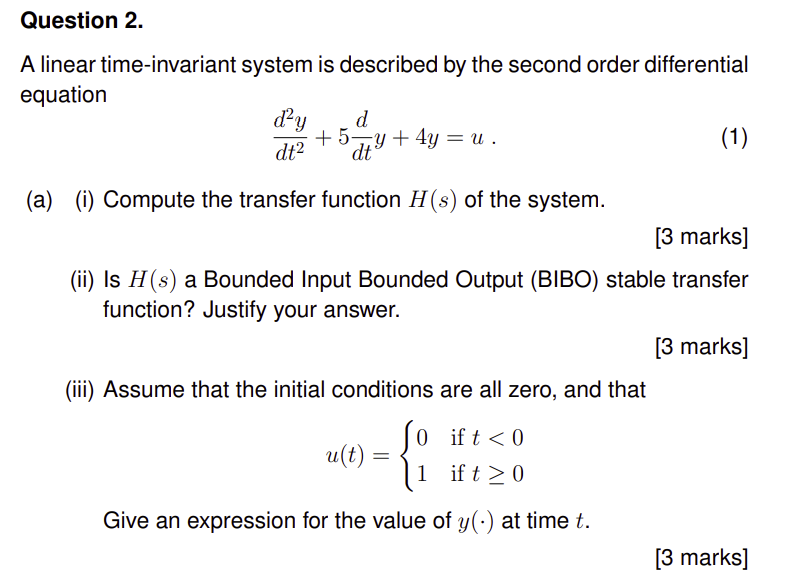Home / Expert Answers / Mechanical Engineering / a-linear-time-invariant-system-is-described-by-the-second-order-differential-pa500

# (Solved): A linear time-invariant system is described by the second order differential ...???????

A linear time-invariant system is described by the second order differential equation $\frac{d^{2} y}{d t^{2}}+5 \frac{d}{d t} y+4 y=u .$ (a) (i) Compute the transfer function $$H(s)$$ of the system. [3 marks] (ii) Is $$H(s)$$ a Bounded Input Bounded Output (BIBO) stable transfer function? Justify your answer. [3 marks] (iii) Assume that the initial conditions are all zero, and that $u(t)=\left\{\begin{array}{ll} 0 & \text { if } t<0 \\ 1 & \text { if } t \geq 0 \end{array}\right.$ Give an expression for the value of $$y(\cdot)$$ at time $$t$$.

We have an Answer from Expert